# Nehari extension problem

Let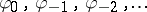be a given sequence of complex numbers. The Nehari extension problem is the problem to find (if possible) allsatisfying the following conditions:

i) theth Fourier coefficientofis equal tofor each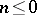;

ii) the norm constraint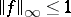holds true. Here,is the norm ofas an element of the Lebesgue function space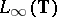and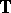is the unit circle. Instead of condition ii) one may require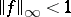, and in the latter case one calls the problem suboptimal.

The Nehari extension problem is not always solvable. In fact (see [a12]), the problem has a solution if and only if the infinite Hankel matrix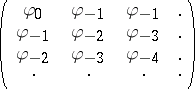induces a bounded linear operatoron, the Hilbert space of all square-summable sequences, such that its operator norm is at most one, i.e.,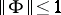. The suboptimal version of the problem is solvable if and only if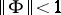. If, either the solution of the Nehari extension problem is unique or there are infinitely many solutions. If, then the problem and its suboptimal version always have infinitely many solutions, which can be parametrized by a fractional-linear mapping.

For the suboptimal case, the set of all solutionsin the Wiener algebra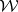, i.e., when one requires additionally that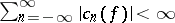, can be described as follows. In this case, it is assumed that the given sequenceis absolutely summable. Let. Then the operators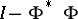andare boundedly invertible on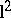, and one can build the following infinite column vectors: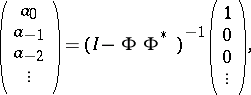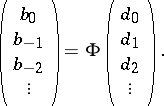Now, consider the functions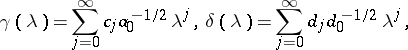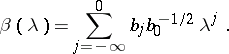Then, each solution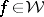of the suboptimal Nehari extension problem for the sequenceis of the form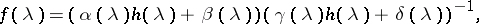(a1)

whereandis an arbitrary element of the Wiener algebrasuch that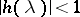for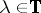and theth Fourier coefficient ofis zero for each. Moreover, (a1) gives a one-to-one correspondence between all suchand all solutions. The central solution, i.e., the solution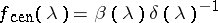, which one obtains when the free parameterin (a1) is identically zero, has a maximum entropy characterization. In fact, it is the unique solutionof the suboptimal Nehari extension problem that maximizes the entropy integral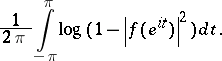The Nehari extension problem has natural generalizations for matrix-valued and operator-valued functions, and it has two-block and four-block analogues. In the matrix-valued case, a superoptimal Nehari extension problem is studied also. In the latter problem the constraint is made not only for the norm, but also for a number of first singular values [a13]. There exist many different approaches to treat the Nehari problem and its various generalizations. For instance, the method of one-step extensions (see [a1]), the commutant-lifting approach (see [a6] and Commutant lifting theorem), the band method (see [a10]), reproducing-kernel Hilbert space techniques (see [a5]), and Beurling–Lax methods in Krein spaces (see [a4] and Krein space). The results are used incontrol theory (see [a8]), and when the data are Fourier coefficients of a rational matrix function, the formulas for the coefficients in the linear fractional representation (a1) can be represented explicitly in state-space form (see [a9] and [a3]).

The Nehari extension problem also has non-stationary versions, in which the role of analytic functions is taken over by lower-triangular matrices. An example is the problem to complete a given lower-triangular array of numbers,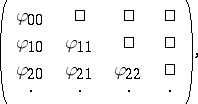to a full infinite matrix such that the resulting operator onis bounded and has operator norm at most one. The non-stationary variants of the Nehari extension problem have been treated in terms of nest algebras [a2]. The main results for the stationary case carry over to the non-stationary case [a11], [a7].

How to Cite This Entry:
Nehari extension problem. Encyclopedia of Mathematics. URL: http://encyclopediaofmath.org/index.php?title=Nehari_extension_problem&oldid=43116
This article was adapted from an original article by I. GohbergM.A. Kaashoek (originator), which appeared in Encyclopedia of Mathematics - ISBN 1402006098. See original article